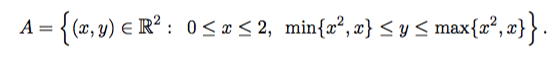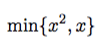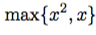• I
DottZakapa
TL;DR Summary
double integralcould please some one explain the inequality on the right?
in particular how should i seeandthanks

Gold Member
You are working with ##0\leq x\leq 2##. If ##0\leq x\leq 1##, then ##\text{min}\{x^2,x\}=x^2## and if ##1\leq x\leq 2##, then ##\text{min}\{x^2,x\}=x## (and other other way around for ##\text{max}##).

DottZakapa
why?

Mentor
why?
Look at the graphs of ##y = x## and ##y = x^2## on the interval [0, 2]. When ##x \in [0, 1]##, which graph is higher? Same question when ##x \in [1, 2]##.

Mentor
The ##min\{x^2,x\}## with x between 0 and 2 inclusively means comparing ##x^2## to ##x## and selecting the lesser number of the two.

As an example, if ##x=-2## then the comparison would be 4 vs -2 and so -2 is the lesser one.

In this case, ##x=0.5## vs ##x^2=0.25## so that ##x^2## is the lesser when ##x## is between 0 and 1.
and for the case, ## x=1.5## vs ##x^2= 2.25## then ##x## is the lesser one when ##x## is between 1 and 2.

DottZakapa
Aw ok now is clear, thanks a lot